## 4.2 Linking views without shiny

### 4.2.1 Motivating examples

As shown in Linking views with shiny, the key attribute provides a way to attach a key (i.e., ID) to graphical elements – an essential feature when making graphical queries. When linking views in plotly outside of shiny, the suggested way to attach a key to graphical elements is via the SharedData class from the crosstalk package (Cheng 2016). At the very least, the new() method for this class requires a data frame, and a key variable. Lets suppose we’re interested in making comparisons of housing sales across cities for a given year using the txhousing dataset. Given that interest, we may want to make graphical queries that condition on a year, so we start by creating a SharedData object with year as the shared key.

library(crosstalk)
sd <- SharedData$new(txhousing, ~year) As far as ggplotly() and plot_ly() are concerned, SharedData object(s) act just like a data frame, but with a special key attribute attached to graphical elements. Since both interfaces are based on the layered grammar of graphics, key attributes can be attached at the layer level, and those attributes can also be shared across multiple views. Figure 3.16 leverages both of these features to link multiple views of median house sales in various Texan cities. As the video shows, hovering over a line in any panel selects that particular year, and all corresponding panels update to highlight that year. The result is an incredibly powerful tool for quickly comparing house sale prices, not only across cities for a given year, but also across years for a given city. p <- ggplot(sd, aes(month, median)) + geom_line(aes(group = year)) + geom_smooth(data = txhousing, method = "gam") + facet_wrap(~ city) ggplotly(p, tooltip = "year") %>% highlight(defaultValues = 2015, color = "red")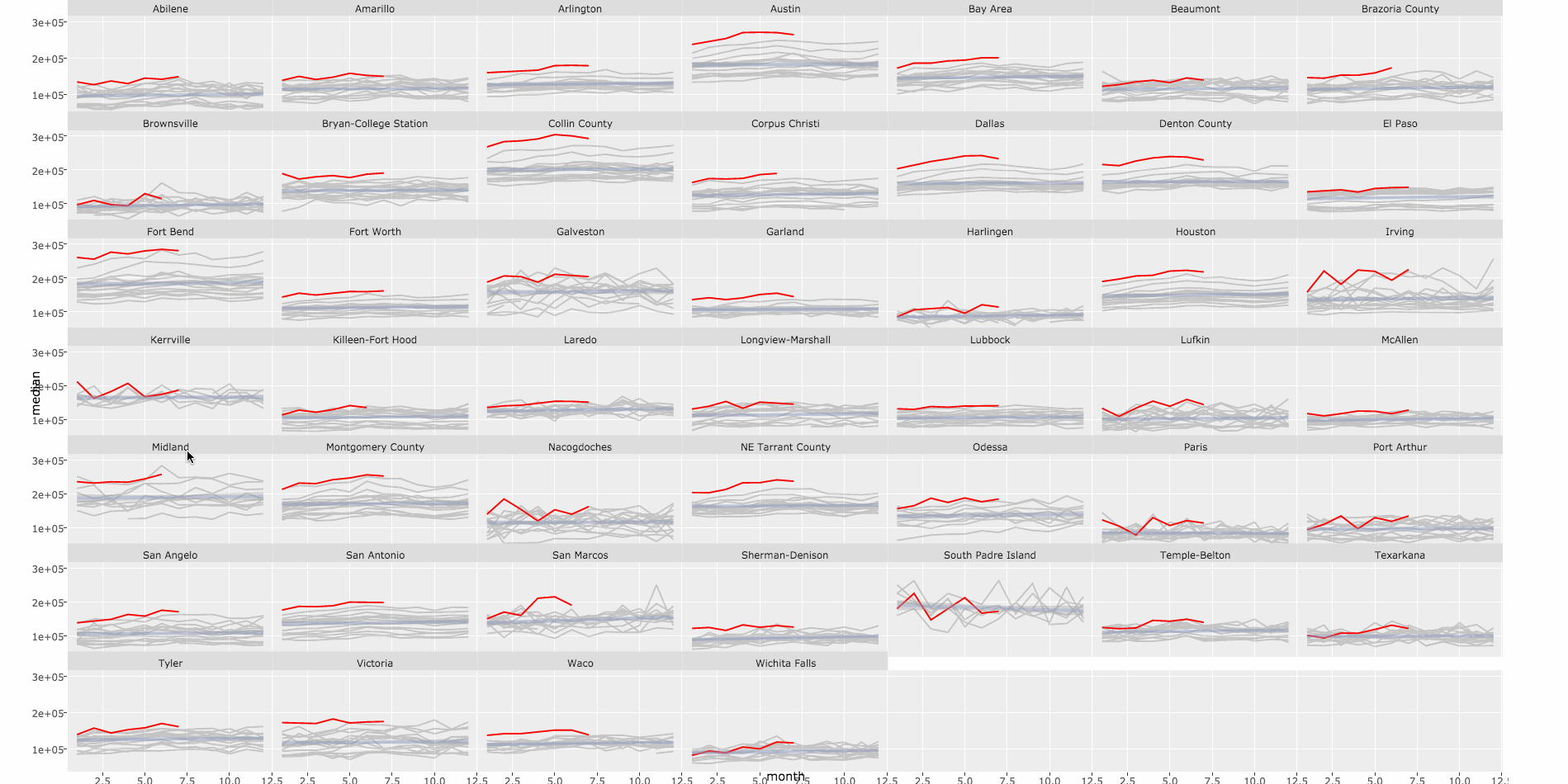Figure 3.16: Monthly median house sales by year and city. Each panel represents a city and panels are linked by year. A video demonstrating the graphical queries can be viewed here Figure 3.16 uses the highlight() function from the plotly package to specify the type of plotly event for triggering a selection (via the on argument), the color of the selection (via the color argument), and set a default selection (via the defaultValues argument). The off argument controls the type of event that clears selections, and if not specified, will default to a sensible event based on the on event (here on='plotly_click') and off='plotly_doubleclick'. The highlight() function can also be used to control Transient versus persistent selection modes, and dynamically control selection colors, which is very useful for making comparisons. Figure 3.17 shows another example of using SharedData objects to link multiple views, this time to enable linked brushing in a scatterplot matrix via the ggpairs() function from the GGally package. As discussed in Scatterplot matrices, the ggpairs() function implements the generalized pairs plot – a generalization of the scatterplot matrix – an incredibly useful tool for exploratory data analysis. Since the Species variable (as discrete variable) is mapped to color in Figure 3.17, we can inspect both correlations, and marginal densities, dependent upon Species type. By adding the brushing capabilities via ggplotly(), we add the ability to examine the dependence between a continuous conditional distribution and other variables. For this type of interaction, a unique key should be attached to each observation in the original data, which is the default behavior of the SharedData object’s new() method when no key is provided. d <- SharedData$new(iris)
p <- GGally::ggpairs(d, aes(color = Species), columns = 1:4)
highlight(ggplotly(p), on = "plotly_selected")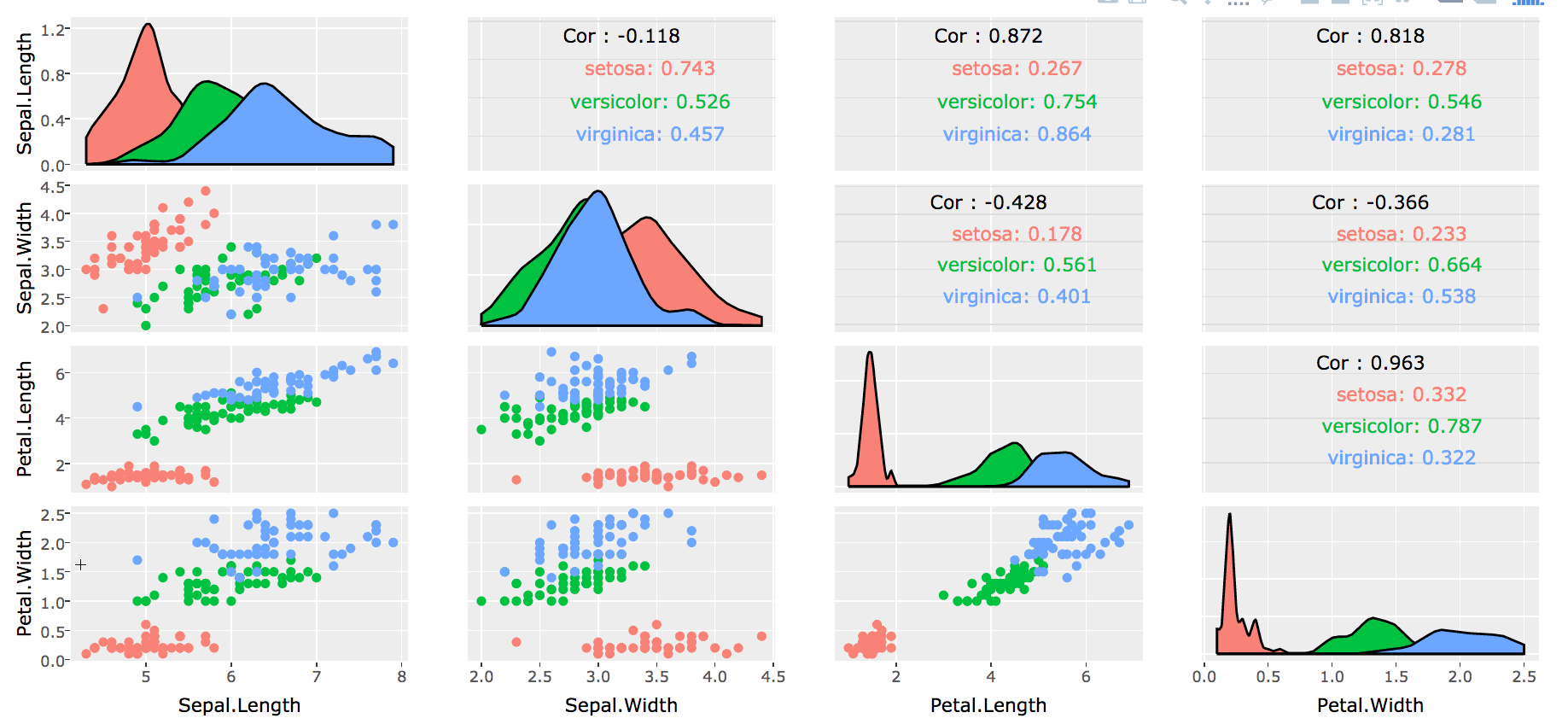Figure 3.17: Brushing a scatterplot matrix via the ggpairs() function in the GGally package. A video demonstrating the graphical queries can be viewed here

When the graphical query is made is 3.17, the marginal densities do not update. This points out one of the weaknesses of implementing multiple linked views without shiny (or some other R backend). The browser knows nothing about the algorithm GGally (or ggplot2) uses to compute a density, so updating the densities in a consistent way is not realistic without being able to call R from the browser. It is true that we could try to precompute densities for every possible selection state, but this does not generally scale well when the number of selection states is large, even as large as Figure 3.17. As discussed briefly in bars & histograms, Boxplots, and 2D distributions, plotly.js does have some statistical functionality that we can leverage to display Dynamic aggregates, but this currently covers only a few types of statistical displays.

### 4.2.2 Transient versus persistent selection

The examples in the previous section use transient selection, meaning that when a value is selected, previous selection(s) are forgotten. Sometimes it is more useful to allow selections to accumulate – a type of selection known as persistent selection. To demonstrate the difference, Figure 3.18 presents two different takes a single view, one with transient selection (on the left) and one with persistent selection (on the right). Both selection modes can be used when linking multiple views, but as Figure 3.18 shows, highlighting graphical elements, even in a single view, can be useful tool to avoid overplotting.

sd <- SharedData$new(txhousing, ~city) p <- ggplot(sd, aes(date, median)) + geom_line() gg <- ggplotly(p, tooltip = "city") # Persistent mode can still be enabled in this case by holding the # shift key when hovering over lines highlight(gg, on = "plotly_hover", dynamic = TRUE) # Persistent mode can be set permanently like so highlight(gg, on = "plotly_hover", dynamic = TRUE, persistent = TRUE)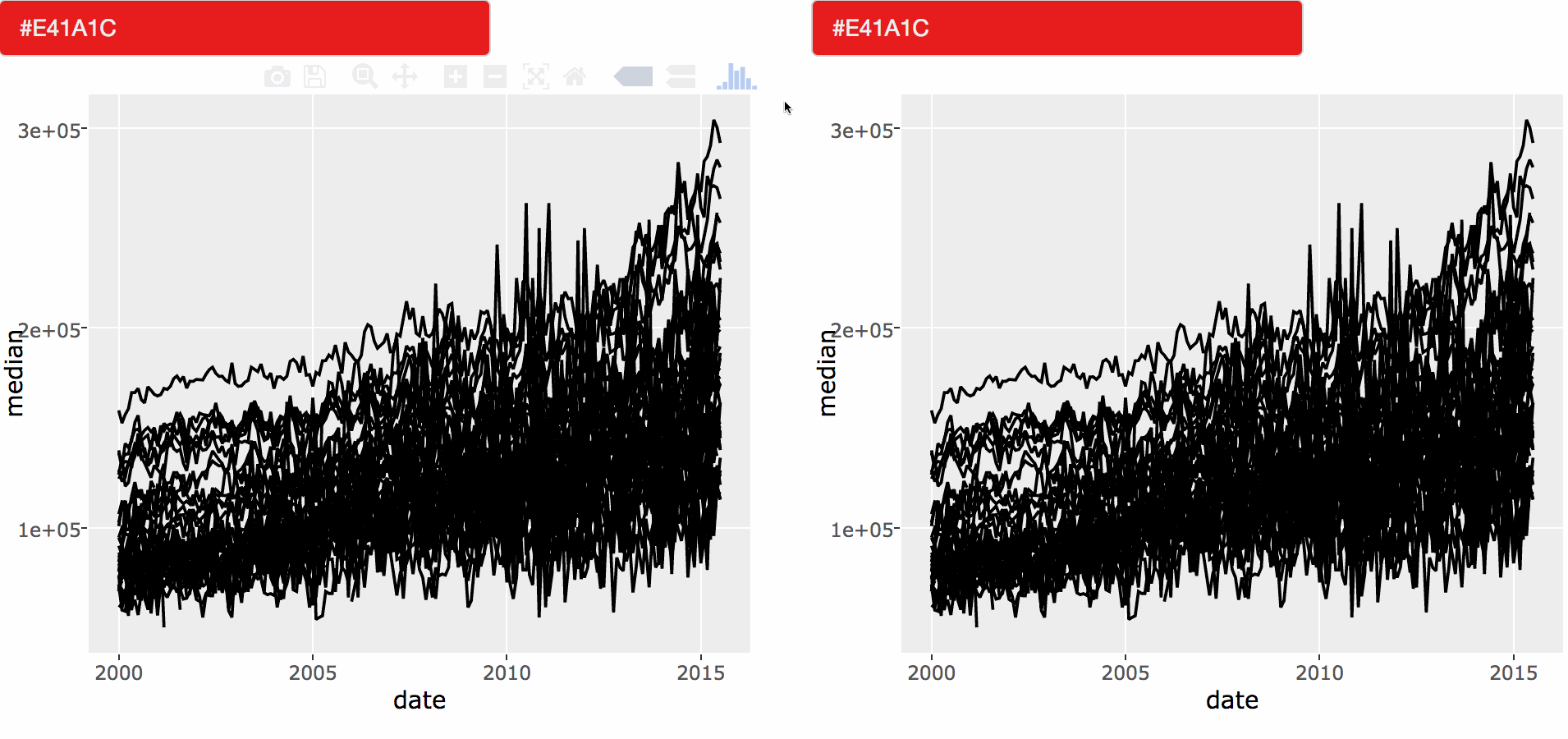Figure 3.18: Highlighting lines with transient versus persistent selection. In the left hand panel, transient selection (the default); and in the right hand panel, persistent selection. The video may be accessed here Figure 3.18 also sets the dynamic argument to TRUE to populate a widget, powered by the colourpicker package (Attali 2016), for dynamically altering the selection color. When paired with persistent selection, this makes for a powerful tool for making comparisons between two selection sets. However, for Figure 3.18, transient selection is probably the better mode for an initial look at the data (to help reveal any structure in missingness or anomalies for a given city), whereas persistent selection is better for making comparisons once have a better idea of what cities might be interesting to compare. ### 4.2.3 Linking with other htmlwidgets Perhaps the most exciting thing about building a linked views framework on top of the crosstalk package is that it provides a standardized protocol for working with selections that other htmlwidget packages may build upon. If implemented carefully, this effectively provides a way to link views between two independent graphical systems – a fairly foreign technique within the realm of interactive statistical graphics. This grants a tremendous amount of power to the analyst since she/he may leverage the strengths of multiple systems in a single linked views analysis. Figure 3.19 shows an example of linked views between plotly and leaflet for exploring the relationship between the magnitude and geographic location of earthquakes. library(plotly) # requires an experimental version of leaflet # devtools::install_github("rstudio/leaflet#346") library(leaflet) sd <- SharedData$new(quakes)

# let plotly & leaflet know this is persistent selection
options(persistent = TRUE)

p <- plot_ly(sd, x = ~depth, y = ~mag) %>%
add_markers(alpha = 0.5) %>%
highlight("plotly_selected", dynamic = TRUE)

map <- leaflet(sd) %>%

bscols(widths = c(6, 6), p, map)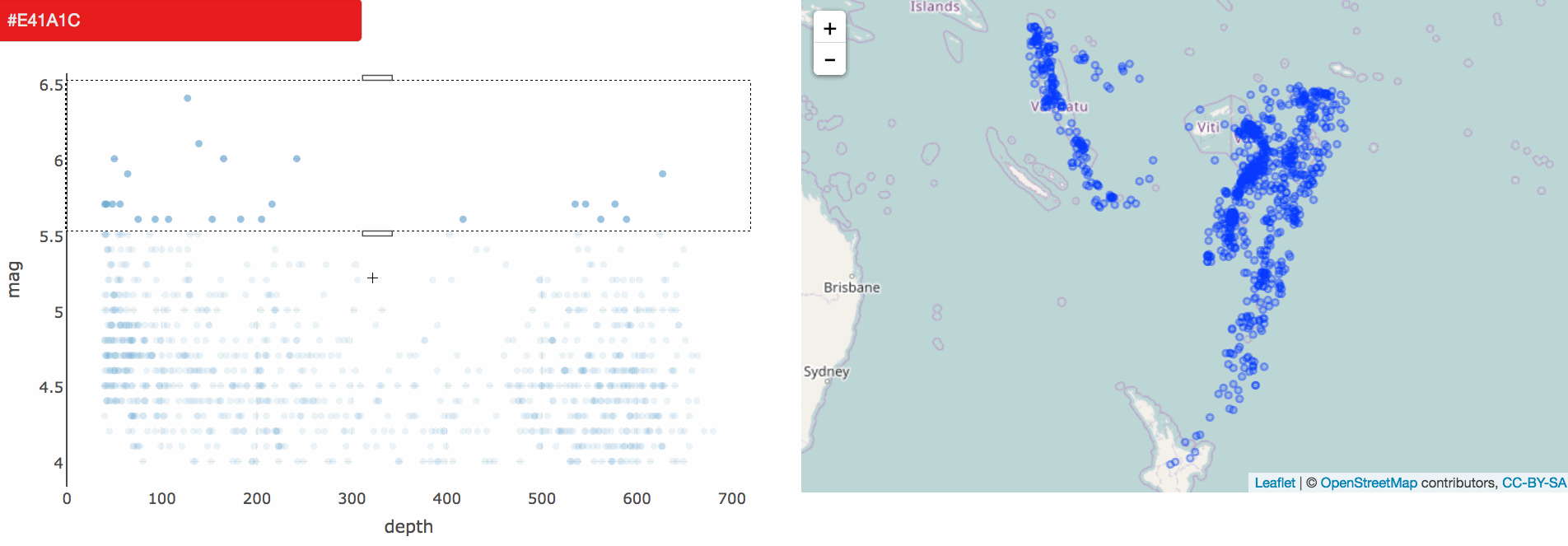Figure 3.19: Linking views between plotly and leaflet to explore the relation between magnitude and geographic location of earthquakes around Fiji. The video may be accessed here

In Figure 3.19, the user first highlights earthquakes with a magnitude of 5 or higher in red (via plotly), then earthquakes with a magnitude of 4.5 or lower, and the corresponding earthquakes are highlighted in the leaflet map. This immediately reveals an interesting relationship in magnitude and geographic location, and leaflet provides the ability to zoom and pan on the map to investigate regions that have a high density of quakes. It’s worth noting that the crosstalk package itself does not provide semantics for describing persistent/dynamic selections, but plotly does inform crosstalk about these semantics which other htmlwidget authors can access in their JavaScript rendering logic.

### 4.2.4 Selection via indirect manipulation

The interactions described thus far in Linking views without shiny is what Cook and Swayne (2007) calls direct manipulation, where the user makes graphical queries by directly interacting with graphical elements. In Figure 3.20, cities are queried indirectly via a dropdown powered by the selectize.js library (B. R. & Contributors 2016). Indirect manipulation is especially useful when you have unit(s) of interest (e.g. your favorite city), but can not easily find that unit in the graphical space. The combination of direct and indirect manipulation is powerful, especially when the interactive widgets for indirect manipulation are synced with direct manipulation events. As shown in Figure 3.20, when cities are queried indirectly, the graph updates accordingly, and when cities are queried directly, the select box updates accordingly. If the time series was linked to other view(s), as it is in the next section, selecting a city via the dropdown menu would highlight all of the relevant view(s).

# Group name is used to populate a title for the dropdown
sd <- SharedData$new(txhousing, ~city, group = "Choose a city") plot_ly(sd, x = ~date, y = ~median) %>% group_by(city) %>% add_lines(text = ~city, hoverinfo = "text") %>% highlight(on = "plotly_hover", persistent = TRUE, selectize = TRUE)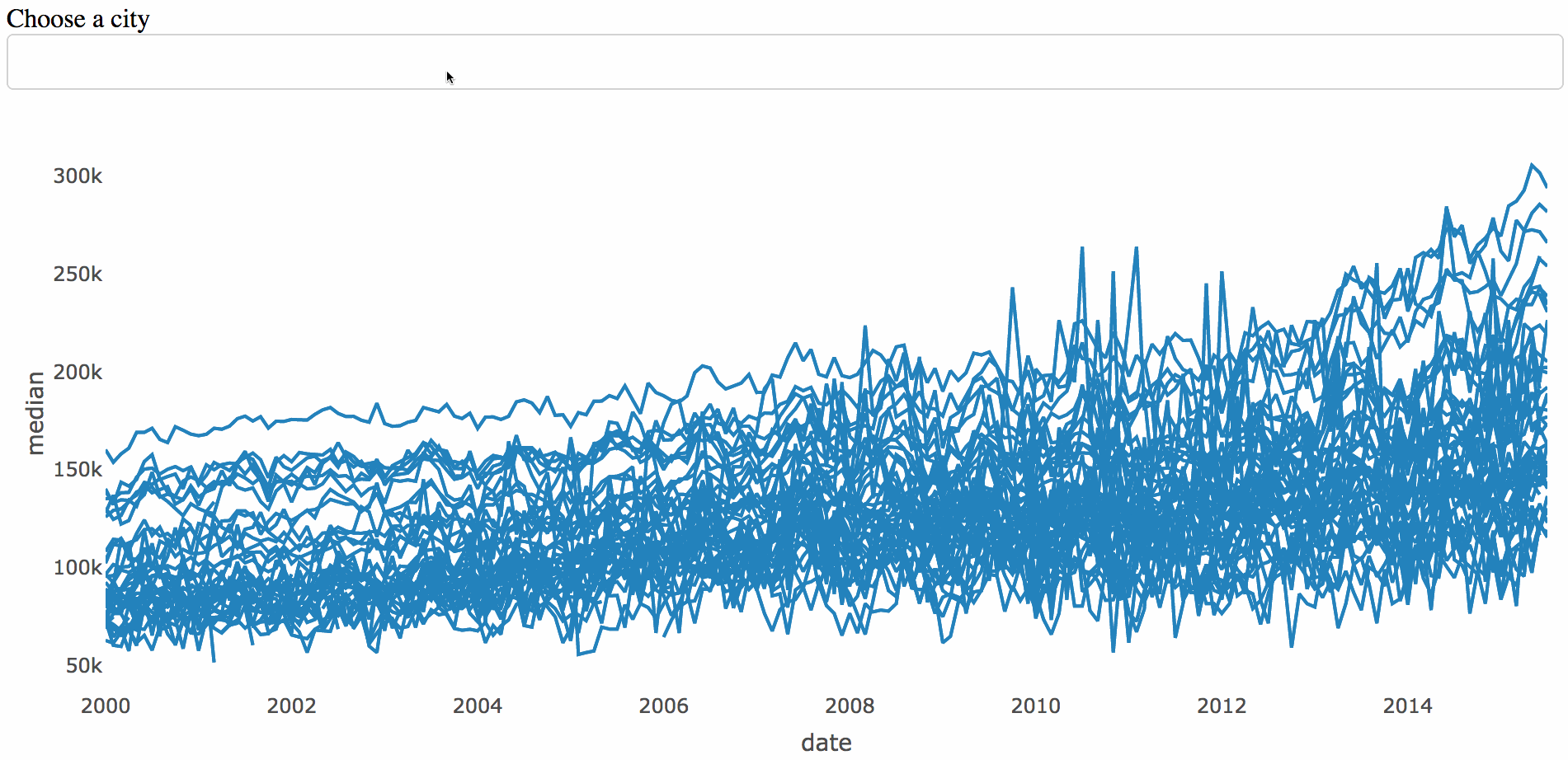Figure 3.20: Selecting cities by indirect manipulation. The video may be accessed here ### 4.2.5 The SharedData plot pipeline Sometimes it is useful to display a summary (i.e., overview) in one view and link that summary to more detailed views. Figure 3.21 is one such example that displays a bar chart of all Texan cities with one or more missing values (the summary) linked with their values over time (the details). By default, the bar chart allows us to quickly see which cities have the most missing values, and by clicking a specific bar, it reveals the relationship between missing values and time for a given city. In cities with the most missing values, data did not start appearing until somewhere around 2006-2010, but for most other cities (e.g., Harlingen, Galveston, Temple-Belton, etc), values started appearing in 2000, but for some reason go missing around 2002-2003.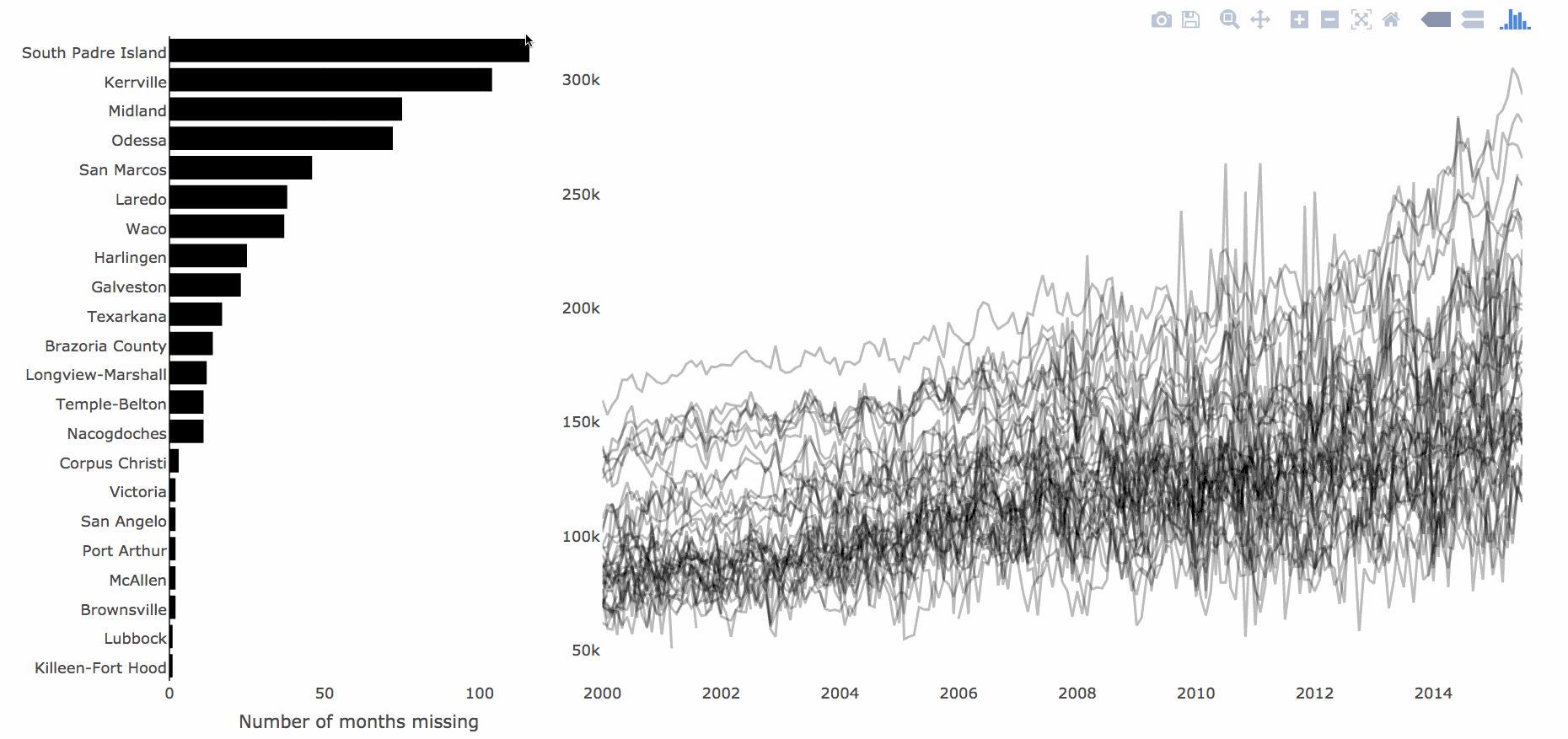Figure 3.21: A bar chart of cities with one or more missing median house sales linked to a time series of those sales over time. The video may be accessed here When implementing linked views like Figure 3.21, it can be helpful to conceptualize a pipeline between a central data frame and the corresponding views. Figure 3.23 is a visual depiction of this conceptual model between the central data frame and the eventual linked views in Figure 3.21. In order to generate the bar chart on the left, the pipeline contains a function for computing summary statistics (the number of missing values per city). On the other hand, the time series does not require any summarization – implying the pipeline for this view is the identity function.Figure 3.22: A diagram of the pipeline between the data and graphics. Since the pipeline from data to graphic is either an identity function or a summarization of some kind, it is good idea to use the most granular form of the data for the SharedData object, and use the data-plot-pipeline to define a pipeline from the data to the plot. As Wickham et al. (2010) writes, a true interactive graphics system is aware of the both the function from the central data object to the graphic, as well as the inverse function (i.e., the function from the graphic back to the central data object). As it currently stands, plotly loses this information when the result is pushed to the web browser, but that does not matter for Figure 3.23 since the pipeline does not need to re-execute statistical summaries of information tied to a user event.15 library(plotly) sd <- highlight_key(txhousing, ~city, "Select a city") base <- plot_ly(sd, color = I("black"), height = 400) %>% group_by(city) p1 <- base %>% summarise(miss = sum(is.na(median))) %>% filter(miss > 0) %>% add_markers(x = ~miss, y = ~forcats::fct_reorder(city, miss), hoverinfo = "x+y") %>% layout( barmode = "overlay", xaxis = list(title = "Number of months missing"), yaxis = list(title = "") ) p2 <- base %>% add_lines(x = ~date, y = ~median, alpha = 0.3) %>% layout(xaxis = list(title = "")) subplot(p1, p2, titleX = TRUE, widths = c(0.3, 0.7)) %>% hide_legend() %>% highlight(dynamic = TRUE, selectize = TRUE) Figure 3.23: An interactive version of Figure 3.21. A selectize widget and a colorpicker widget have been added for making comparisons. Try it by first clicking on a bar, then change the brush color, then hold the shift key (enabling persistent selection) while clicking on a different bar. ### 4.2.6 Dynamic aggregates As discussed in the plotly cookbook, there are a number of way to compute statistical summaries in the browser via plotly.js (e.g., add_histogram(), add_boxplot(), add_histogram2d(), and add_histogram2dcontour()). When linking views with the plotly package, we can take advantage of this functionality to display aggregated views of selections. Figure 3.24 shows a basic example of brushing a scatterplot to select cars with 10-20 miles per gallon, then a 5 number summary of the corresponding engine displacement is dynamically computed and displayed as a boxplot. d <- SharedData$new(mtcars)
scatterplot <- plot_ly(d, x = ~mpg, y = ~disp) %>%

subplot(
plot_ly(d, y = ~disp, color = I("black")) %>%
scatterplot, shareY = TRUE
) %>% highlight("plotly_selected")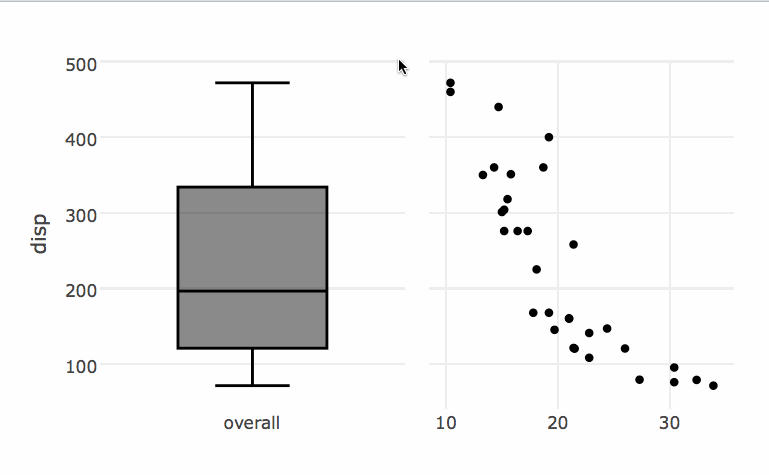Figure 3.24: Dynamically populating a boxplot reflecting brushed observations

Figure 3.24 is very similar to Figure 3.25, but uses add_histogram() to link to a bar chart of the number of cylinders rather than a boxplot of engine displacement. By brushing to select cars with a low engine displacement, we can see that (obviously) displacement is related with to the number of cylinders.

p <- subplot(
plot_ly(d, x = ~factor(vs)) %>% add_histogram(color = I("black")),
scatterplot
)

# Selections are actually additional traces, and, by default,
# plotly.js will try to dodge bars placed under the same category
p %>%
layout(barmode = "overlay") %>%
highlight("plotly_selected")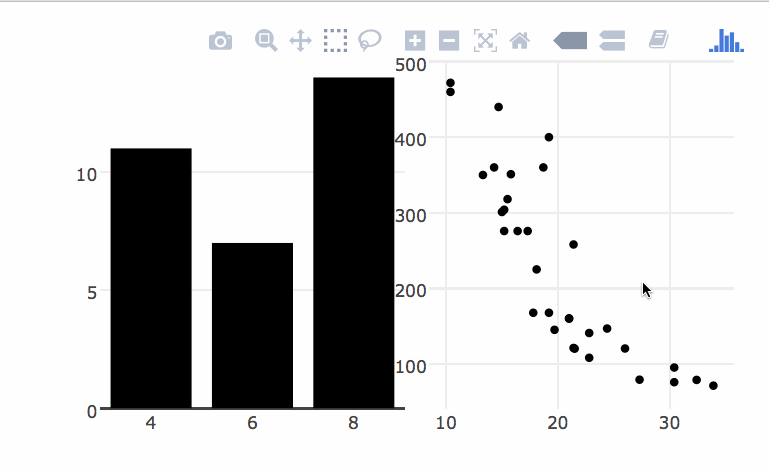Figure 3.25: Dynamically populating a bar chart reflecting brushed observations

### 4.2.7 Nested selections

#### 4.2.7.1 Grouped selections via ggplotly()

In statistical graphics, it is quite common for a graphical element to be tied to multiple observations. For example, a line representing the fitted values from a linear model is inherently connected to the observations used to fit the model. In fact, any graphical summary (e.g. boxplot, histogram, density, etc.) can be linked back the original data used to derive them. Especially when comparing multiple summaries, it can be useful to highlight group(s) of summaries, as well as the raw data that created them. Figure 3.26 uses ggplotly()’s built-in support for linking graphical summaries with raw data to enable highlighting of linear models.16 Furthermore, notice how there are actually two levels of selection in Figure 3.26 – when hovering over a single point, just that point is selected, but when hovering over a fitted line, all the observations tied to that line are selected.

# if you don't want to highlight individual points, you could specify
# class as the key variable here, instead of the default (rownames)
m <- SharedData$new(mpg) p <- ggplot(m, aes(displ, hwy, colour = class)) + geom_point() + geom_smooth(se = FALSE, method = "lm") ggplotly(p) %>% highlight("plotly_hover")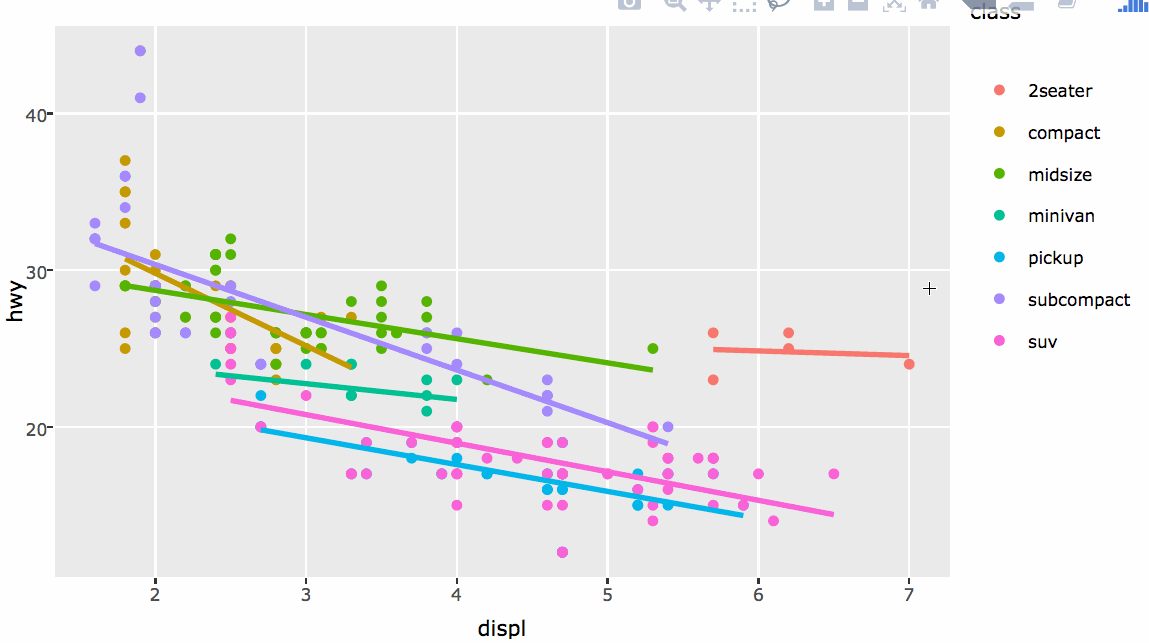Figure 3.26: Engine displacement versus highway miles per gallon by class of car. The linear model for each class, as well as the individual observations, can be selected by hovering over the line of fitted values. An individual observation can also be selected by hovering over the relevant point. At first, it might not seem as though “nested” selections are that useful. After all, the highlighting of individual points in Figure 3.26 does not supply us with any additional information. However, applying this same idea to multiple views of the same observations can be quite useful. Figure 3.27 demonstrates a useful application via the ggnostic() function from the GGally package (Schloerke et al. 2016). This function produces a matrix of diagnostic plots from a fitted model object, with different diagnostic measures in each row, and different explanatory variables in each column. Figure 3.27 shows the default display for a linear model, which includes residuals (resid), estimates of residual standard deviation when a particular observation is excluded (sigma), diagonals from the projection matrix (hat), and cooks distance (cooksd). # for better tick labels mtcars$am <- dplyr::recode(mtcars$am, 0 = "automatic", 1 = "manual") # choose a model by AIC stepping backwards mod <- step(lm(mpg ~ ., data = mtcars), trace = FALSE) # produce diagnostic plots, coloring by automatic/manual pm <- GGally::ggnostic(mod, mapping = aes(color = am)) # ggplotly() automatically adds rownames as a key if none is provided ggplotly(pm) %>% highlight("plotly_click")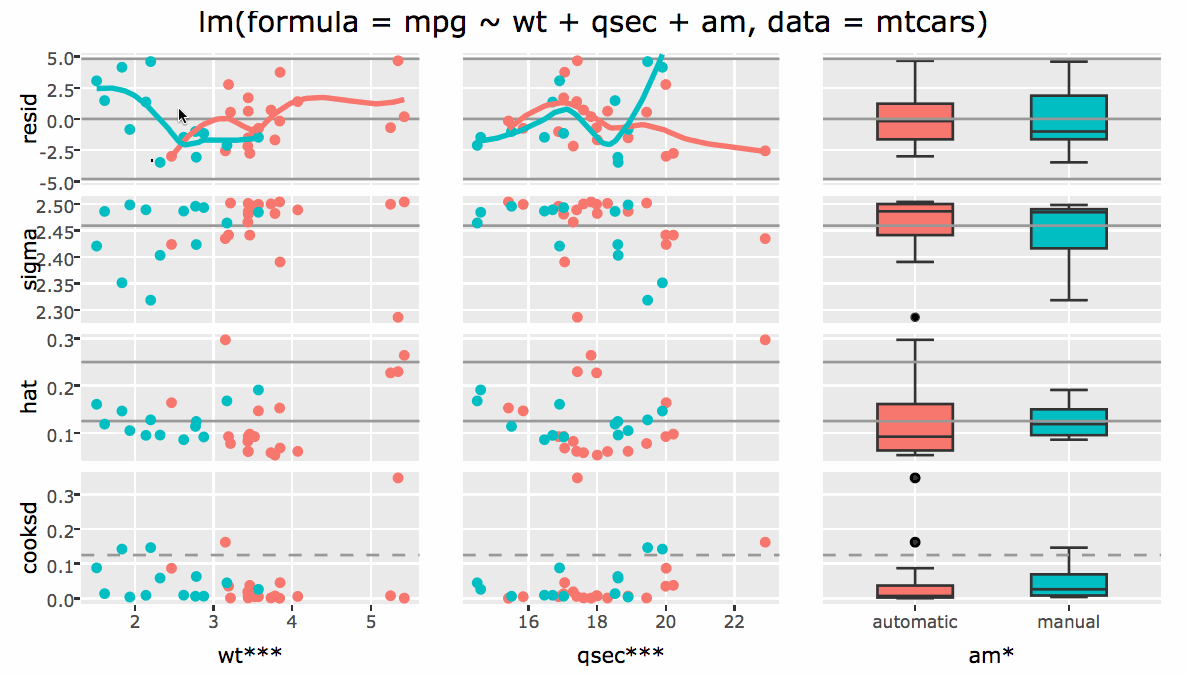Figure 3.27: Using nested selections to highlight numerous diagnostics from different regions of the design matrix. Injecting interactivity into ggnostic() via ggplotly() enhances the diagnostic plot in at least two ways. Coloring by a factor variable in the model allows us to highlight that region of the design matrix by selecting a relevant statistical summary, which can help avoid overplotting when dealing with numerous factor levels. For example, in Figure 3.27, the user first highlights diagnostics for cars with manual transmission (in blue), then cars with automatic transmission (in red). Perhaps more widely useful is the ability to highlight individual observations since most of these diagnostics are designed to identify highly influential or unusual observations. In Figure 3.27, there is one observation with a noticeably high value of cooksd, which suggests the observation has a large influence on the fitted model. Clicking on that point highlights its corresponding diagnostic measures, plotted against each explanatory variable. Doing so makes it obvious that this observation is influential since it has a unusually high response/residual in a fairly sparse region of the design space (i.e., it has a pretty high value of wt) and removing it would significantly reduce the estimated standard deviation (sigma). By comparison, the other two observations with similar values of wt have a response value very close to the overall mean, so even though their value of hat is high, their value of sigma is low. Highlighting groups via fitted lines is certainly useful, but it is not the only way to highlight groups via a graphical summary. In fact, anytime a key variable is supplied to ggplot2 layer with a non-identity statistic, it automatically attaches all the unique key values tied to all the observations that went into creating the group – enabling linked selections between raw and aggregated forms of the data. For example, Figure 3.28 links several density estimates back to the original data, and by clicking on a particular density estimate, it highlights all of the observations associated with that group. Yet again, this is an effective strategy to combat overplotting by bring groups of interests to the foreground of the graphic. m <- SharedData$new(mpg)
p1 <- ggplot(m, aes(displ, fill = class)) + geom_density()
p2 <- ggplot(m, aes(displ, hwy, fill = class)) + geom_point()
subplot(p1, p2) %>% highlight("plotly_click") %>% hide_legend()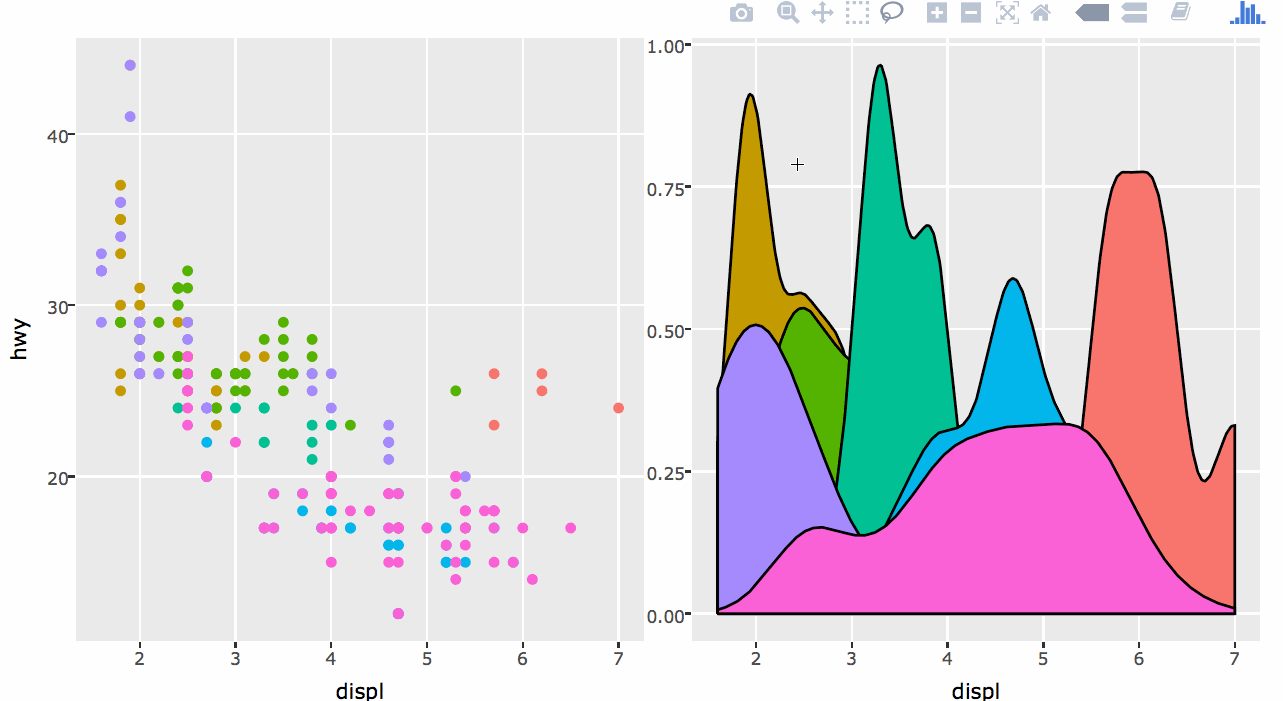Figure 3.28: Clicking on a density estimate to highlight all the raw observations that went into that estimate.

#### 4.2.7.2 Hierarchical selection

In the previous section, we leveraged ggplotly()’s ability to attach multiple key values to a graphical object to perform “grouped selections” on statistical objects (e.g., selecting a density estimate selects all the corresponding observations). In all those examples, groups are distinct (specifically, no one observation can be an input to more than one group), so what happens when groups are not distinct? Under the hood, plotly considers each graphical mark to be a “group”, in the sense that a set of element(s)/value(s) can be associated with each mark. Given selected mark(s) (i.e. groups of interest), it considers any subset of the selection(s) to be match, leading to a notion of hierarchical selection. For a simple example, suppose I have 4 (x, y) pairs, and each pair is associated with a different set of categorical values:

# data frames do support list columns,
# but tibble::tibble() provides a nicer API for this...
d <- data.frame(x = 1:4, y = 1:4)
d$key <- lapply(1:4, function(x) letters[seq_len(x)]) d #> x y key #> 1 1 1 a #> 2 2 2 a, b #> 3 3 3 a, b, c #> 4 4 4 a, b, c, d Suppose point (3, 3) is selected – implying the set $$\{ a, b, c \}$$ is of interest – what key sets should be considered a match? The most sensible approach is to match any subsets of the selected set, so for example, this key set would match the sets $$\{ a \}$$, $$\{ b \}$$, $$\{ c \}$$, $$\{ a, b \}$$, $$\{ a, c \}$$, and $$\{ b, c \}$$. This leads to a type of selection I will refer to as hierarchical selection. Figure 3.29 provides a visual demo of this example in action: SharedData$new(d, ~key) %>%
plot_ly(x = ~x, y = ~y) %>%
highlight("plotly_selected") %>%
layout(dragmode = "lasso")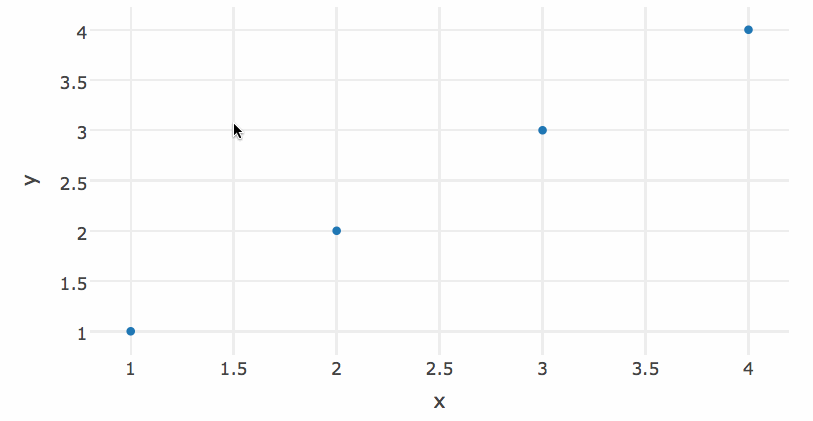Figure 3.29: A simple example of hierarchial selection

Another way to think of hierarchical selection is to select all the “children” of a given “parent” node, which has a natural extension to dendrograms as 3.30 demonstrates. The demo, demo("tour-basic", package = "plotly"), has an example of linking a dendrogram with a grand tour – a powerful technique for interactively diagnosing classification models.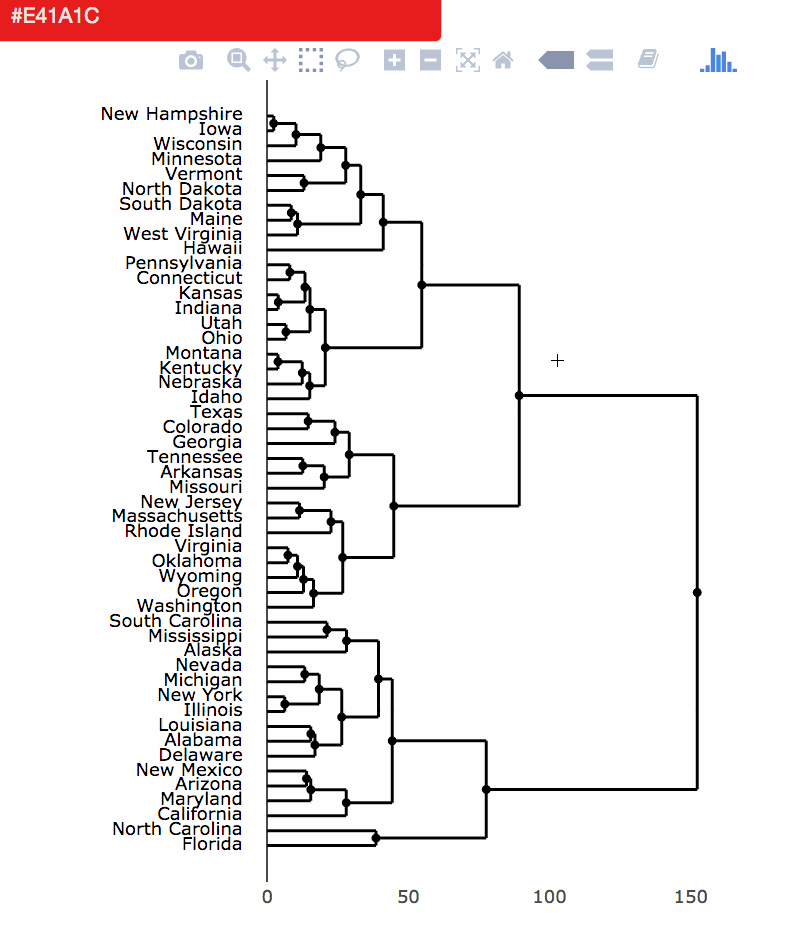Figure 3.30: Leveraging hierarchical selection and persistent brushing to paint branches of a dendrogram.

### 4.2.8 More examples

The plotly package bundles a bunch of demos that illustrate all the options available when linking views without shiny via crosstalk’s SharedData class and plotly’s highlight() function. To list all the examples, enter demo(package = "plotly") into your R prompt, and pick a topic (e.g., demo("highlight-intro", package = "plotly")).

### 4.2.9 Limitations

In terms of linking views without shiny, the biggest limitation is the lack of ability to perform statistical aggregations in real time based on user queries. For example, Figure 3.36 could be improved by layering on a linear model specific to the user selection, to make it easier to compare and track those relationships over time. In this case, one may want to opt into linking views with shiny to trigger the execution of R code in response to a user query. While this is a fundamental limitation of the system design, there are also a number of current limitations from an implementation perspective.

### References

Cheng, Joe. 2016. Crosstalk: Inter-Widget Interactivity for Html Widgets.

Attali, Dean. 2016. Colourpicker: A Colour Picker Widget for Shiny Apps, Rstudio, R-Markdown, and ’Htmlwidgets’. https://CRAN.R-project.org/package=colourpicker.

Cook, Dianne, and Deborah F. Swayne. 2007. Interactive and Dynamic Graphics for Data Analysis : With R and Ggobi. Use R ! New York: Springer. http://www.ggobi.org/book/.

Contributors, Brian Reavis &. 2016. “Selectize Is an Extensible jQuery-Based Custom <select> Ui Control.” 2016. https://github.com/selectize/selectize.js.

Wickham, Hadley, Michael Lawrence, Dianne Cook, Andreas Buja, Heike Hofmann, and Deborah F Swayne. 2010. “The Plumbing of Interactive Graphics.” Computational Statistics, April, 1–7.

Schloerke, Barret, Jason Crowley, Di Cook, Francois Briatte, Moritz Marbach, Edwin Thoen, Amos Elberg, and Joseph Larmarange. 2016. GGally: Extension to ’Ggplot2’.

1. Since dplyr semantics translate to SQL primitives, you could imagine a system that translates a data-plot-pipeline to SQL queries, and dynamically re-executes within the browser via something like SQL.js (O. L. & Contributors 2016).

2. Strictly speaking, as long as a key is provided to a ggplot2 layer with a non-identity statistic, ggplotly() will nest keys within group.